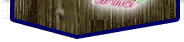home about pictures reference trade links

### Graphics matrix operations:

3-D points are generally stored in four element vectors, defined as:
```[X, Y, Z, W]
```
...where X, Y, and Z are the point 3-D coordinates, and W is the 'weight', and is used to normalise the result after an operation, multiplying each element by 1/W so that W ends equal to 1.

Points can be moved around by matric multiplication with 4X4 transformation matrices. Multiplying a vector with a matric produces a new vector, which is the transformed point. Standard transformation matrices are:

```Identity (does not transform point):

[ 1   0   0   0 ]
[ 0   1   0   0 ]
[ 0   0   1   0 ]
[ 0   0   0   1 ]

Translate (move along X, Y, Z axes):

[ 1   0   0   0 ]
[ 0   1   0   0 ]
[ 0   0   1   0 ]
[ Tx  Ty  Tz  1 ]

Scale (translate to larger or smaller coordinates):

[ Sx  0   0   0 ]
[ 0   Sy  0   0 ]
[ 0   0   Sz  0 ]
[ 0   0   0   1 ]

Rotate (around X, Y, or Z axis by angle U):
Axis X:            Axis Y:            Axix Z:

[ 1   0   0   0 ]  [cosU 0 -sinU 0 ]  [cosU sinU 0   0 ]
[ 0 cosU sinU 0 ]  [ 0   1   0   0 ] [-sinU cosU 0   0 ]
[ 0-sinU cosU 0 ]  [sinU 0  cosU 0 ]  [ 0   0    1   0 ]
[ 0   0   0   1 ]  [ 0   0   0   1 ]  [ 0   0    0   1 ]

Perspective (d is the distance of "eye" behind "screen"):

[ 1   0   0   0 ]
[ 0   1   0   0 ]
[ 0   0   1   0 ]
[ 0   0  1/d  0 ]

```
Transformation matrices can be combined by multiplying them together, so a single matrix can be use to shift, rotate, and scale a point in a single operation. Other 3-D operations using vectors are also frequently used, such as to determine intersection points or the reflection of light rays.

Previous Page